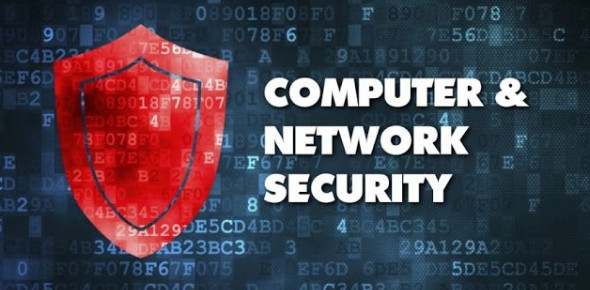# Quiz: Computer And Network Security Practice Questions!

20 Questions | Total Attempts: 105Settings.

• 1.
A process of making the encrypted text readable again.
• A.

Decryption

• B.

Encryption

• C.

Network Security

• D.

Information Hiding

• 2.
A small program that changes the way a computer operates.
• A.

Worm

• B.

Trojan

• C.

Bomb

• D.

Virus

• 3.
Ram chooses 7 and 11 as two prime numbers. He chooses 13 as the value of e. The ciphertext obtained using RSA algorithm is 26. What is the planintext?
• 4.
Cryptanalysis is used __________
• A.

To find some insecurity in a cryptographic scheme

• B.

To increase the speed

• C.

To encrypt the data

• D.

To make new ciphers

• 5.
Which one of the following is a cryptographic protocol used to secure HTTP connection?
• A.

Stream control transmission protocol (SCTP)

• B.

Transport layer security (TLS)

• C.

• D.

Resource reservation protocol

• 6.
If end to end connection is done at a network or IP level, and if there are 10 hosts, then what is the number of keys required?
• 7.
. When a hash function is used to provide message authentication, the hash function value is called to as:
• 8.
Using Rabin cryptosystem with p=23 and q=7 Encrypt P=24 to find ciphertext. The Cipher text is
• 9.
In the DES algorithm the round key is __________ bit and the Round Input is ____________bits.
• A.

48, 32

• B.

64,32

• C.

56, 24

• D.

32, 32

• 10.
In tunnel mode, IPSec protects the ______
• A.

• B.

IP trailer

• C.

Entire IP packet

• D.

• 11.
Which component is included in IP security?
• A.

• B.

• C.

Internet key Exchange (IKE)

• D.

All of the mentioned

• 12.
A hash function guarantees the integrity of a message. It guarantees that the message has not be
• A.

Replaced

• B.

Over view

• C.

Changed

• D.

Violated

• 13.
The responsibility of certification authority for digital signature is to authenticate
• A.

Hash function used

• B.

Private key of the user

• C.

Public key of the user

• D.

Key used in AES

• 14.
For a client-server authentication, the client requests from the KDC a ________ for access to a specific asset.
• 15.
Message _______ means that the receiver is ensured that the message is coming from the intended sender, not an imposter.
• A.

Confidentiality

• B.

Integrity

• C.

Authentication

• D.

All

• 16.
A digital certificate system
• A.

Uses third-party CAs to validate a user's identity.

• B.

Uses digital signatures to validate a user's identity.

• C.

Uses tokens to validate a user's identity.

• D.

Are used primarily by individuals for personal correspondence.

• 17.
In case of public key cryptography, X adds a digital signature K to message M, encrypts < M, K >, and sends it to Y, where it is decrypted. Choose the correct sequences of keys is used for the operations?
• A.

Encryption: X’s private key followed by Y’s private key; Decryption: X’s public key followed by Y’s public key

• B.

Encryption: X’s private key followed by Y’s public key; Decryption: X’s public key followed by Y’s private key

• C.

Encryption: X’s public key followed by Y’s private key; Decryption: Y’s public key followed by X’s private key

• D.

Encryption: X’s private key followed by Y’s public key; Decryption: Y’s private key followed by X’s public key

• 18.
How many keys are required for two people to communicate via a cipher?
• A.

Two key for symmetric ciphers, two keys for asymmetric ciphers

• B.

One key for symmetric ciphers and asymmetric ciphers

• C.

One key for symmetric ciphers, two keys for asymmetric ciphers

• D.

Two key for symmetric ciphers, one keys for asymmetric ciphers

• 19.
What will be the plain text corresponding to ciphered text “rmw ” if an affine cipher is used with key values as a=5, b=10?
• A.

Csk

• B.

Kkr

• C.

Srt

• D.

Msd

• 20.
X=3mod7, X=3mod13, X=0mod12. The value of X is__________
Related TopicsBack to top